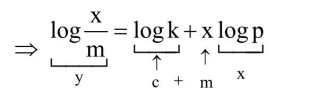# The mass of gas adsorbed, x, per unit mass of adsorbate,

Question:

The mass of gas adsorbed, $x$, per unit mass of adsorbate, $\mathrm{m}$, was measured at various pressures, p. A graph between $\log \frac{x}{m}$

and $\log p$ gives a straight line with slope equal to 2 and the intercept equal to $0.4771$. The value of $\frac{x}{m}$ at a pressure

of $4 \mathrm{~atm}$ is : (Given $\log 3=0.4771$ )

Solution:

$\frac{x}{m}=k p^{x}$.....(1)Given $\mathrm{c}=\log \mathrm{k}=0.4771$ or $\mathrm{k}=3$

slope $x=2$

put in eq. (1) $\frac{\mathrm{x}}{\mathrm{m}}=3 \times(4)^{2} \Rightarrow 48$